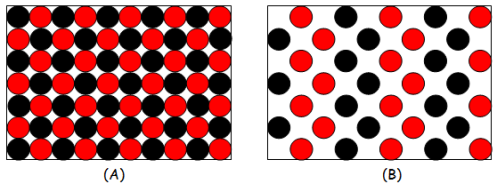# Density: Definition, Formula & Practice Problems

An error occurred trying to load this video.

Try refreshing the page, or contact customer support.

Coming up next: Glycosidic Bond: Definition & Formation

### You're on a roll. Keep up the good work!

Replay
Your next lesson will play in 10 seconds
• 0:04 What Is Density?
• 1:12 Calculating Density
• 2:29 Lesson Summary

Want to watch this again later?

Timeline
Autoplay
Autoplay
Speed

#### Recommended Lessons and Courses for You

Lesson Transcript
Instructor: Richard Cardenas

Richard Cardenas has taught Physics for 15 years. He has a Ph.D. in Physics with a focus on Biological Physics.

In this lesson, you'll learn about density, the way in which density can be calculated from mass and volume, and that density of substances are constant. You'll get to calculate densities of various materials in different situations.

## What Is Density?

Let's say we have three identical cubes. Each side of the cube has a 1.0-centimeter length. One cube is made out of lead, the second cube is made out of aluminum, and the third cube is made out of wood. If I asked you which cube would be the most dense of the three cubes, you will most likely say the one made out of lead. Simple enough. But what, just exactly, is density?

Density is defined as the ratio between mass and volume or mass per unit volume. It is a measure of how much stuff an object has in a unit volume (cubic meter or cubic centimeter). Mass is a measure of how much stuff an object contains, and volume is the measure of how much space an object occupies in three-dimensional space. This figure shows a dense object versus a less dense object:As you can see in the figure, the dense object (A) has more stuff than the less dense object (B). Both objects are shown to occupy the same space.

## Calculating Density

So, how do we find the density of an object? As you saw, you need two pieces of information in order to calculate density: mass (in grams or kilograms), and volume (in cubic centimeter or cubic meter). The formula for density is:Going back to the three cubes in the beginning of the lesson, let us calculate the densities of the three objects. Let's say we have 11.3 grams of lead, 2.7 grams of aluminum, and 0.67 grams of wood (pinewood). The densities are calculated as mass per unit volume (1 cubic centimeter).

To unlock this lesson you must be a Study.com Member.

### Register to view this lesson

Are you a student or a teacher?

### Unlock Your Education

#### See for yourself why 30 million people use Study.com

##### Become a Study.com member and start learning now.
Back
What teachers are saying about Study.com

### Earning College Credit

Did you know… We have over 160 college courses that prepare you to earn credit by exam that is accepted by over 1,500 colleges and universities. You can test out of the first two years of college and save thousands off your degree. Anyone can earn credit-by-exam regardless of age or education level.